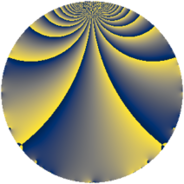# Properties

 Label 3630.2.cLevel $3630$ Weight $2$ Character orbit 3630.c Rep. character $\chi_{3630}(2179,\cdot)$ Character field $\Q$ Dimension $110$ Sturm bound $1584$

# Related objects

## Defining parameters

 Level: $$N$$ $$=$$ $$3630 = 2 \cdot 3 \cdot 5 \cdot 11^{2}$$ Weight: $$k$$ $$=$$ $$2$$ Character orbit: $$[\chi]$$ $$=$$ 3630.c (of order $$2$$ and degree $$1$$) Character conductor: $$\operatorname{cond}(\chi)$$ $$=$$ $$5$$ Character field: $$\Q$$ Sturm bound: $$1584$$

## Dimensions

The following table gives the dimensions of various subspaces of $$M_{2}(3630, [\chi])$$.

Total New Old
Modular forms 840 110 730
Cusp forms 744 110 634
Eisenstein series 96 0 96

## Trace form

 $$110q - 110q^{4} + 4q^{5} - 2q^{6} - 110q^{9} + O(q^{10})$$ $$110q - 110q^{4} + 4q^{5} - 2q^{6} - 110q^{9} + 2q^{10} - 4q^{14} - 2q^{15} + 110q^{16} + 16q^{19} - 4q^{20} - 12q^{21} + 2q^{24} + 10q^{25} - 4q^{26} + 4q^{30} - 16q^{31} - 4q^{34} + 28q^{35} + 110q^{36} + 4q^{39} - 2q^{40} - 36q^{41} - 4q^{45} - 8q^{46} - 102q^{49} + 24q^{50} + 4q^{51} + 2q^{54} + 4q^{56} + 20q^{59} + 2q^{60} - 52q^{61} - 110q^{64} + 44q^{65} - 8q^{69} + 40q^{70} - 72q^{71} - 4q^{74} + 24q^{75} - 16q^{76} - 16q^{79} + 4q^{80} + 110q^{81} + 12q^{84} + 44q^{85} - 24q^{86} - 4q^{89} - 2q^{90} - 24q^{91} - 16q^{94} - 2q^{96} + O(q^{100})$$

## Decomposition of $$S_{2}^{\mathrm{new}}(3630, [\chi])$$ into newform subspaces

The newforms in this space have not yet been added to the LMFDB.

## Decomposition of $$S_{2}^{\mathrm{old}}(3630, [\chi])$$ into lower level spaces

$$S_{2}^{\mathrm{old}}(3630, [\chi]) \cong$$ $$S_{2}^{\mathrm{new}}(30, [\chi])$$$$^{\oplus 3}$$$$\oplus$$$$S_{2}^{\mathrm{new}}(55, [\chi])$$$$^{\oplus 8}$$$$\oplus$$$$S_{2}^{\mathrm{new}}(110, [\chi])$$$$^{\oplus 4}$$$$\oplus$$$$S_{2}^{\mathrm{new}}(165, [\chi])$$$$^{\oplus 4}$$$$\oplus$$$$S_{2}^{\mathrm{new}}(330, [\chi])$$$$^{\oplus 2}$$$$\oplus$$$$S_{2}^{\mathrm{new}}(605, [\chi])$$$$^{\oplus 4}$$$$\oplus$$$$S_{2}^{\mathrm{new}}(1210, [\chi])$$$$^{\oplus 2}$$$$\oplus$$$$S_{2}^{\mathrm{new}}(1815, [\chi])$$$$^{\oplus 2}$$